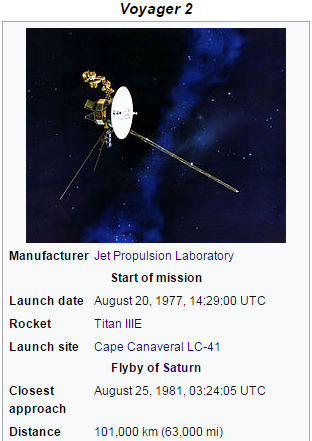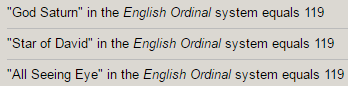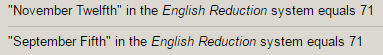## Cipher## Jan 6, 2017

### Voyager 1 and 2 - beautiful coding from NASA as alwaysLaunch site - Cape Canaveral LC-41 - (LC stands for 'Space Launch Complex' = 74), in Reduction system, LC = 3 3
"Cape Canaveral" = 3+1+7+5+3+1+5+1+22+5+9+1+3 = 66 with V exception (initials - C.C. - 33 - "Thirty-three" = 66)

Voyager 2 was launched 16 days before Voyager 1 (Sixteen = 33), I don't know why they decided to first launch Voyager 2 then 1Launched on August 20th 1977, a date that leaves 133 days (Big Bang Theory = 133, Evolution = 133, Government = 133)234+432 = 666

8/20/1977 - 8+2+0+1+9+7+7 = 34
8/20/1977 - 8+20+1+9+7+7 = 52 (Voyager Two = 52)
8/20/77 = 105 (Zionism = 105, Masonry = 105, American Dream = 105, Nine Eleven = 105)

Closest approach to Saturn was on 8/25/1981 (8+25 = 33)8/25/1981 - 8+2+5+1+9+8+1 = 34 (just like launch date numerology)
8/25/1981 - 8+25+1+9+8+1 = 52 (again same as launch date numerology)
8/25/1981 - 8+25+19+81 = 133 (launch date left 133 days in the year), in my last post I mentioned that 133 is one of 3 special numbers that sum to 1331 in Jewish system

I found something really interesting in regards to their take offVoyager 1 took off at 12:56 - (12*60 + 56 = 776th minute of the day)
Voyager 2 took off at 14:29 - (14*60 + 29 = 869th minute of the day)
869 - 776 = 93  (Voyager = 93, Saturn = 93)

Both 'space probes' were launched by "Titan IIIE" rocket which was retired after launching Voyager 1 'into space'First flight was on 2/11 - 211 - 47th prime number in year 1974, also it is 42nd day in the year (Outer Space = 42, Gas Giant = 42, Freemason = 42, Zionism = 42, History = 42)

2/11/1974 - 2+11+1+9+7+4 - 34 (34th prime is 139 - Freemasonry = 139, Global Economy = 139, Elite = 139 Jewish G.)
2/11/74 - 2+11+74 = 87But interestingly from First to Last flight of Titan rocket is 1303 days a lot like 133 (Government = 133, White house = 133, God Bless America = 133, Alien Agenda = 133 in Jewish systemThe rocket was used from 1974 to 1977, Jesus = 74, Christ = 77, Hexagram = 77, Judaism = 77 (77th triangular number is 3003)

Retired after dropping Voyager 1 into space on it's Seventh flightSeventh flight = 74 (S exception)I forgot to mention the manufacturer of Voyager 1 and 2 - "Jet Propulsion Laboratory" = 1+5+2 + 7+9+6+7+3+3+10+9+6+5 + 3+1+2+6+9+1+2+6+9+7 = 119 (S exception)
Saturn = 93+26 =  119 (Francis Bacon Gematria)Voyager One = 127 E. Ordinal (31st prime number)
Voyager One = 22+6+7+1+7+5+9 + 6+5+5 = 73 - V exception (73 is 21st prime number, Saturn = 21)

Voyager One launched on 9/5/1977 - 9+5+1+9+7+7 = 38
9/5/1977 = 9+5+19+77 = 110 (Jet Propulsion Laboratory = 110)Launch and closest approach to Saturn have the same date gematriaClosest approach was at 77000 miles, again 77 goes well with overall coding of 33, 74, 93

11/12/1980 - 11+12+1+9+8+0 = 41 (13th prime number, launched from LC-41, Hexagram = 77/41)
From 11/12/1980 to the date when Voyager Two was at it's closest approach to Saturn is exactly 41 weeks287 days is also interesting336+633 = 969 (Speed of Saturn = 9.69 km/s)